Mathematical Logic as based on the Theory of Types

BY   BERTRAND  RUSSELL

Published in: American Journal of Mathematics, vol.30(1908), pp. 222-262
Transcribed into hypertext by Burtzev B.I., Jul., 07, 2003
e-mail: bbi-math@narod.ru,   site: http://bbi-math.narod.ru/

VI.

Primitive Ideas and Propositions of Symbolic Logic.

CZ.The primitive ideas required in symbolic logic appear to be the following seven:
DA.(1) Any propositional function of a variable x or of several variables x, y, z, . . . This will be denoted by φx or φ(x, y, z, . .)
DB.(2) The negation of a proposition. If p is the proposition, its negation will be denoted by ~p.
DC.(3) The disjunction or logical sum of two propositions; i.e., Уthis or that.Ф If p, q are the two propositions, their disjunction will be denoted by p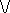q 19)
DD.(4) The truth of any value of a propositional function; i.e., of φx where x is not specified.
DE.(5) The truth of all values of a propositional function. This is denoted by (x).φx or (x):φx or whatever larger number of dots may be necessary to bracket off the proposition.20) In (x).φx, x is called an apparent variable, whereas when φx is asserted, where x is not specified, x is called a real variable.
DF.(6) Any predicative function of an argument of any type; this will be represented by φ!x or φ!α or φ!R, according to circumstances. A predicative function of x is one whose values are propositions of the type next above that of x, if x is an individual or a proposition, or that of values of x if x is a function. It may be described as one in which the apparent variables, if any, are all of the same type as x or of lower type ; and a variable is of lower type than x if it can significantly occur as argument to x, or as argument to an argument to x, etc.
DG.(7) Assertion; i.e., the assertion that some proposition is true, or that any value of some propositional function is true. This is required to distinguish a proposition actually asserted from one merely considered, or from one adduced as hypothesis to some other. It will be indicated by the sign У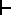Ф prefixed to what is asserted, with enough dots to bracket off what is asserted.21)
DH.Before proceeding to the primitive propositions, we need certain definitions. In the following definitions, as well as in the primitive propositions, the letters p, q, r are used to denote propositions.

p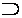q.=.~pq   Df.

DI.This definition states that УpqФ (which is read Уp implies qФ) is to mean Уp is false or q is true.Ф I do not mean to affirm that УimpliesФ can not have any other meaning, but only that this meaning is the one which it is most convenient to give to УimpliesФ in symbolic logic. In a definition, the sign of equality and the letters УDfФ are to be regarded as one symbol, meaning jointly Уis defined to mean.Ф The sign of equality without the letters УDfФ has a different meaning, to be defined shortly.

p.q.=.~(~p~q)   Df.

DJ.This defines the logical product of two propositions p and q, i.e., Уp and q are both true.Ф The above definition states that this is to mean: УIt is false that either p is false or q is false.Ф Here again, the definition does not give the only meaning which can be given to Уp and q are both true,Ф but gives the meaning which is most convenient for our purposes.

p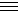q.=.pq.qp   Df.

DK.That is, Уpq,Ф which is read Уp is equivalent to qФ means Уp implies q and q implies p;Ф whence, of course, it follows that p and q are both true or both false.

(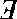x).φx.=.~{(x).~φx}   Df.

DL.This defines Уthere is at least one value of x for which φx is true.Ф We define it as meaning Уit is false that φx is always false.Ф

x = y. = :(φ):φ!x.!y   Df.

DM.This is the definition of identity. It states that x and y are to be called identical when every predicative function satisfied by x is satisfied by y. It follows from the axiom of reducibility that if x satisfies ψx, where ψ is any function, predicative or non-predicative, then y satisfies ψy.
DN.The following definitions are less important, and are introduced solely for the purpose of abbreviation.
 (x, y).φ(x, y) = :(x:(y).φ(x, y) Df. (x, y).φ(x, y) = :(x):(y).φ(x, y) Df. φx.x .ψx = :(x):φxψx Df. φx.x .ψx = :(x):φx .. ψx Df. φ(x, y).x, y .ψ(x, y) : = :(x, y):φ(x, y).. ψ(x, y) Df.,
and so on for any number of variables.
DO.The primitive propositions required are as follows. (In 2, 3, 4, 6, 6, and 10, p, q, r stand for propositions.)
(1) A proposition implied by a true premise is true.
(2): pp.. p.
(3): q..pq.
(4): pq.. pq.
(5): p(qr)..q(pr) .
(6): .qr.: pq..pr .
(7): (x).φx..φy;
i.e., Уif all values of φx^ are true, then φy is true, where φy is any value.Ф22)
DP.(8) If φy is true, where φy is any value of φx^, then (x).φx is true. This can not be expressed in our symbols; for if we write Уφy..(x).φxФ that means Уφy implies that all values of φx^ are true, where y may have any value of the appropriate type,Ф which is not in general the case. What we mean to assert is: УIf, however y is chosen, φy is true, then (x).φx is true,Ф whereas what is expressed by Уφy..(x).φxФ is: УHowever y is chosen, if φy is true, then (x).φx is true,Ф which is quite a different statement, and in general a false one.
DQ.(9): (x).φx..φa, where a is any definite constant.
DR.This principle is really as many different principles as there are possible values of a. I.e., it states that, e.g., whatever holds of all individuals holds of Socrates; also that it holds of Plato; and so on. It is the principle that a general rule may be applied to particular cases; but in order to give it scope, it is necessary to mention the particular cases, since otherwise we need the principle itself to assure us that the general rule that general rules may be applied to particular cases may be applied (say) to the particular case of Socrates. It is thus that this principle differs from (7); our present principle makes a statement about Socrates, or about Plato, or some other definite constant, whereas (7) made a statement about a variable.
DS.The above principle is never used in symbolic logic or in pure mathematics, since all our propositions are general, and even when (as in Уone is a numberФ) we seem to have a strictly particular case, this turns out not to be so when closely examined. In fact, the use of the above principle is the distinguishing mark of applied mathematics. Thus, strictly speaking, we might have omitted it from our list.

DT.(10): (x).pφx.: p..(x).φx;

i.e., Уif Сp or φx is always true,Т then either p is true, or φx is always true.Ф
DU.(11) When f(φx) is true whatever argument x may be, and F(φy) is true whatever possible argument y may be, then {f(φx).F(φx)} is true whatever possible argument x may be.
DV.This is the axiom of the Уidentification of variables.Ф It is needed when two separate propositional functions are each known to be always true, and we wish to infer that their logical product is always true. This inference is only legitimate if the two functions take arguments of the same type, for otherwise their logical product is meaningless. In the above axiom, x and y must be of the same type, because both occur as arguments to φ.
DW.(12) If φx.φx.ψx is true for any possible x, then ψx is true for any possible x.
DX.This axiom is required in order to assure us that the range of significance of ψx, in the case supposed, is the same as that of φx.φxψx..ψx; both are in fact the same as that of φx. We know, in the case supposed, that ψx is true whenever φx.φxψx and φx.φxψx..ψx are both significant, but we do not know, without an axiom, that ψx is true whenever ψx is significant. Hence the need of the axiom.
DY.Axioms (11) and (12) are required, e.g., in proving
(x).φx:(x).φxψx:.(x).ψx

By (7) and (11),:.(x).φx:(x).φxψx::φy.φyψy

whence by (12),:.(x).φx:(x).φxψx:: ψy,

whence the result follows by (8) and (10).
DZ.(13):.(f):.(x):φx.. f ! x.
EA.This is the axiom of reducibility. It states that, given any function φx^, there is a predicative function f ! x^ such that f ! x is always equivalent to φx. Note that, since a proposition beginning with У(f)Ф is, by definition, the negation of one beginning with У(f),Ф the above axiom involves the possibility of considering Уall predicative functions of x.Ф If φx is any function of x, we can not make propositions beginning with У(φ)Ф or У(f)Ф since we can not consider Уall functions,Ф but only Уany functionФ or Уall predicative functions.Ф
EB.(14):.(f):.(x, y):φ(x, y).. f ! (x, y).
EC.This is the axiom of reducibility for double functions.
ED.In the above propositions, our x and y may be of any type whatever. The only way in which the theory of types is relevant is that (11) only allows us to identify real variables occurring in different contents when they are shown to be of the same type by both occurring as arguments to the same function, and that, in (7) and (9), y and a must respectively be of the appropriate type for arguments to φz^. Thus, for example, suppose we have a proposition of the form (φ). f ! ! x ), which is a second-order function of x. Then by (7),:(φ). f ! ! z^, x).. f ! ! z^, x),

where ψ ! z^ is any first-order function. But it will not do to treat (φ). f ! ! z^, x) as if it were a first-order function of x, and take this function as a possible value of ψ ! z^ in the above. It is such confusions of types that give rise to the paradox of the liar.
EE.Again, consider the classes which are not members of themselves. It is plain that, since we have identified classes with functions,23) no class can be significantly said to be or not to be a member of itself; for the members of a class are arguments to it, and arguments to a function are always of lower type than the function. And if we ask: УBut how about the class of all classes? Is not that a class, and so a member of itself?Ф, the answer is twofold. First, if Уthe class of all classesФ means Уthe class of all classes of whatever type,Ф then there is no such notion. Secondly, if Уthe class of all classesФ means Уthe class of all classes of type t,Ф then this is a class of the next type above t, and is therefore again not a member of itself.
EF.Thus although the above primitive propositions apply equally to all types, they do not enable us to elicit contradictions. Hence in the course of any deduction it is never necessary to consider the absolute type of a variable; it is only necessary to see that the different variables occurring in one proposition are of the proper relative types. This excludes such functions as that from which our fourth contradiction was obtained, namely: УThe relation R holds between R and S.Ф For a relation between R and S is necessarily of higher type than either of them, so that the proposed function is meaningless.

 Notes
 Contents: I.    The Contradictions. II.   All and Any III.  The Meaning and Range of Generalized Propositions. IV.   The Hierarchy of Types. V.    The Axiom of Reducibility. VI.   Primitive Ideas and Propositions of Symbolic Logic. VII.  Elementary Theory of Classes and Relations. VIII. Descriptive Functions. IX.   Cardinal Numbers. X.    Ordinal Numbers. —одержание: I.    ѕарадоксы. II.   “¬се, каждый”   и   “любой, произвольный” III. —мысл и область применимости обобще... IV. V. VI. VII. VIII. IX. X.